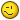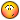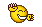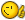# Draw circles in multiple points## Recommended PostsHi,

I want to make a script who draw circles in multiple Points. The Points are automaticly made in a extension to autocad. All the Points are in same layers. It´s around 200 to 1000 Points in the drawing.

My first issue is to find a commando who draw circles, with basepoints in all Points.

Does anyone know the appropriate command for me?

Regards,

Emil

##### Share on other sitesThis is what I use.

```(defun c:ic (/ a b c r d n p d1 d2 e1 cl oerr osn)

(setq osn (getvar "osmode"))
(setvar "cmdecho" 0)

(setq cl (getvar "clayer"))
(if c
()
(setq c 1.0)
)
(princ "\n Circle diameter < ")
(princ c)
(princ " > ? ")
(setq b (getdist))
(if (= b nil)
(setq b c)
(setq c b)
)
(setq r (/ b 2))
(princ "\n Select nodes ")
(setq a (ssget '((0 . "POINT"))))
(setq d (sslength a))
(setq d1 d)
(repeat d
(setq d2 (1- d1))
(setq n (ssname a d2))
(setq e1 (entget n))
(setq p (cdr (assoc 10 e1)))
(entmake (list (cons 0 "CIRCLE")
(cons 6 "BYLAYER")
(cons 8 cl)
(cons 10 p)
(cons 40 r)
(cons 210 (list 0.0 0.0 1.0))
)
)

(entdel n)
(setq d1 d2)
)
(setvar "osmode" osn)
(princ)
)```

##### Share on other sitesMaybe a slight simplification of code

```(setq d (sslength a))
(setq d1 d)
(repeat d
(setq d2 (1- d1))
(setq n (ssname a d2))
(setq e1 (entget n))

[color=lime](repeat (setq d2 (sslength a)))[/color]
[color=lime]    (setq e1 (entget (ssname a (setq d2 (- d2 1)))))[/color]

```

##### Share on other sitesAnother one

```(defun c:test (/ a b i)
(if (and (setq a (ssget '((0 . "point"))))
(setq b (getdist "\nSpecify Circle Radius : "))
)
(repeat (setq i (sslength a))
(entmakex
(list (cons 0 "CIRCLE")
(assoc 10 (entget (ssname a (setq i (1- i)))))
(cons 40 b)
)
)
)
)
(princ)
)```

Edited by satishrajdev
##### Share on other sites@Satish, Note that:

`(cons 10 (cdr (assoc 10 <list>))) == (assoc 10 <list>)`##### Share on other sitesI remember someone wrote an algorithm for spacing circles within range (not sure if it was Marko).

Can't seem to find it right now.

##### Share on other sites@Satish, Note that:

`(cons 10 (cdr (assoc 10 <list>))) == (assoc 10 <list>)`Thanks you sir. I need to be more focused on thisBTW I have updated the code.

##### Share on other sites

• 2 weeks later...Thanks so much.

It works good!

Another one
```(defun c:test (/ a b i)
(if (and (setq a (ssget '((0 . "point"))))
(setq b (getdist "\nSpecify Circle Radius : "))
)
(repeat (setq i (sslength a))
(entmakex
(list (cons 0 "CIRCLE")
(assoc 10 (entget (ssname a (setq i (1- i)))))
(cons 40 b)
)
)
)
)
(princ)
)```

##### Share on other sites##### Share on other sitesFWIW, here's another version which will retain the original properties of the points, and will account for points constructed in any UCS plane:

```(defun c:p2c ( / c i r s x )
(if (and (setq s (ssget '((0 . "POINT"))))
(progn
(initget 6)
(setq r (getdist "\nSpecify circle radius: "))
)
)
(repeat (setq i (sslength s))
(setq x (reverse (entget (ssname s (setq i (1- i)))))
c (assoc 10 x)
)
(entmake
(subst '(0 . "CIRCLE") '(0 . "POINT")
(subst (cons 10 (trans (cdr c) 0 (cdr (assoc 210 x)))) c
(reverse
(cons (cons 40 r)
(vl-remove-if '(lambda ( x ) (member (car x) '(50 102 360))) x)
)
)
)
)
)
)
)
(princ)
)```

##### Share on other sitesThats some interesting list manipulation Lee!I would remove GCs -1 5 and 100 aswell (just in case). Although it seems that entmake/x ignores them:

```_\$ (entmake (list (cons 0 "POINT") (cons 5 "MyHandle") (cons 100 "AcDbLWPolyline") (cons 62 1) (cons 10 (getpoint))))
((0 . "POINT") (5 . "MyHandle") (100 . "AcDbLWPolyline") (62 . 1) (10 -38.7621 156.008 0.0))
_\$ (entget (entlast))
((-1 . <Entity name: 7ff678604cc0>) (0 . "POINT") (330 . <Entity name: 7ff6786039f0>) (5 . "24C") (100 . "AcDbEntity") (67 . 0) (410 . "Model") (8 . "0") (62 . 1) (100 . "AcDbPoint") (10 -38.7621 156.008 0.0) (210 0.0 0.0 1.0) (50 . 0.0))
_\$ ```

##### Share on other sitesThats some interesting list manipulation Lee!Thanks!

I would remove GCs -1 5 and 100 aswell (just in case).

I agree with removing the subclass markers in general, as these will cause issues when generating the following entities:

```ATTRIB (Multiline)
ELLIPSE
HATCH
LWPOLYLINE
MTEXT
SPLINE
XLINE         ```

However, it is not necessary to remove DXF groups -1 & 5, as these are always ignored.

## Join the conversation

You can post now and register later. If you have an account, sign in now to post with your account.
Note: Your post will require moderator approval before it will be visible.Reply to this topic...

×   Pasted as rich text.   Restore formatting

Only 75 emoji are allowed.